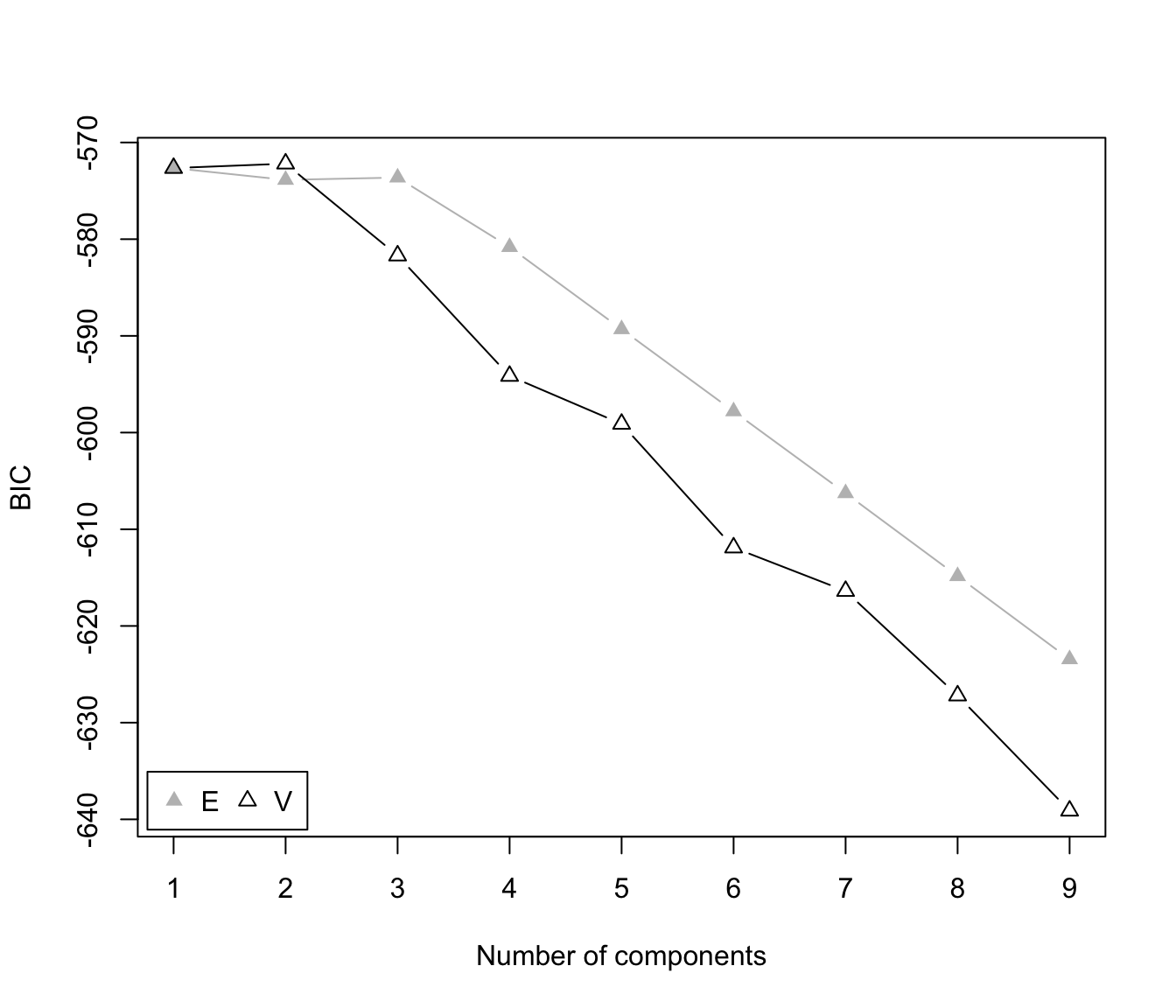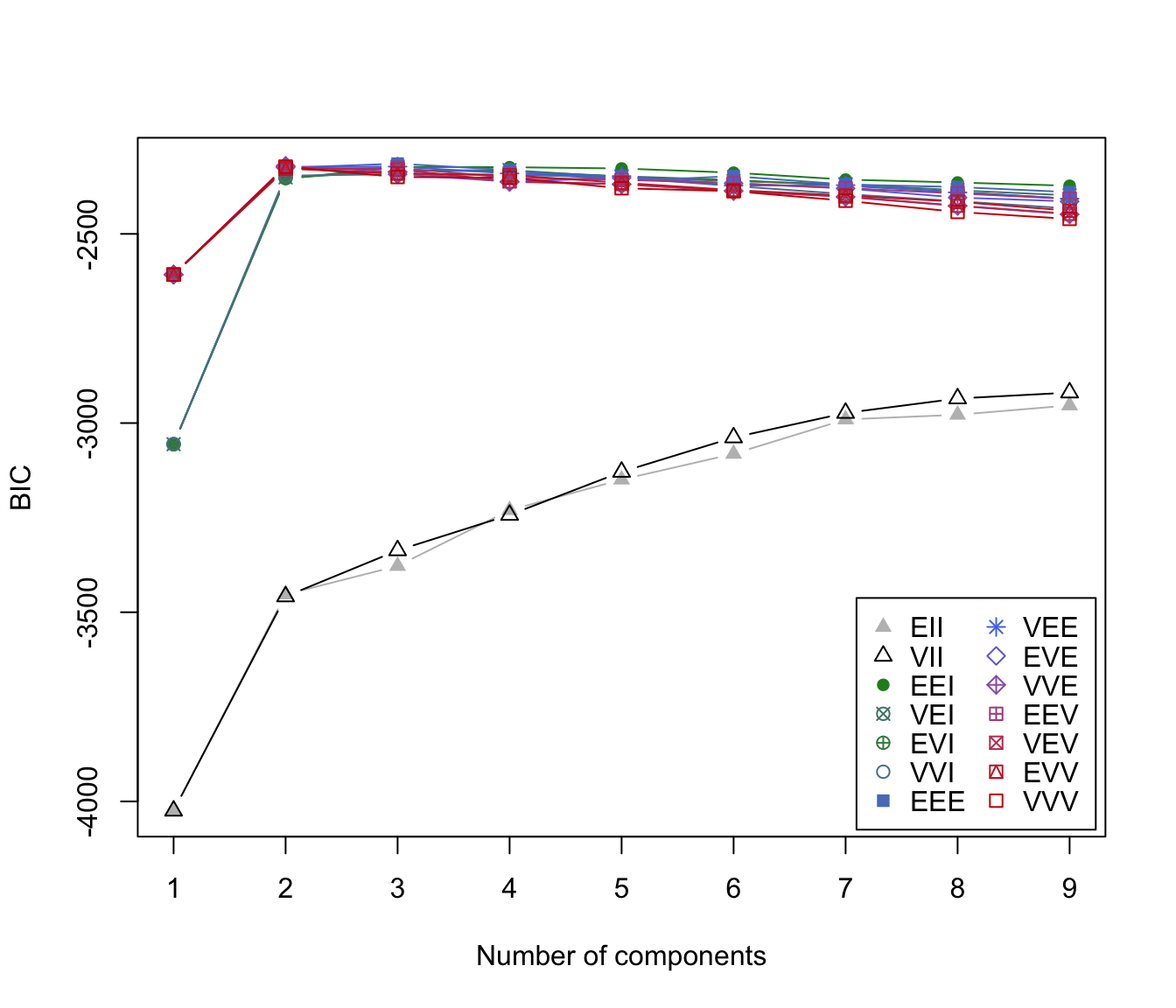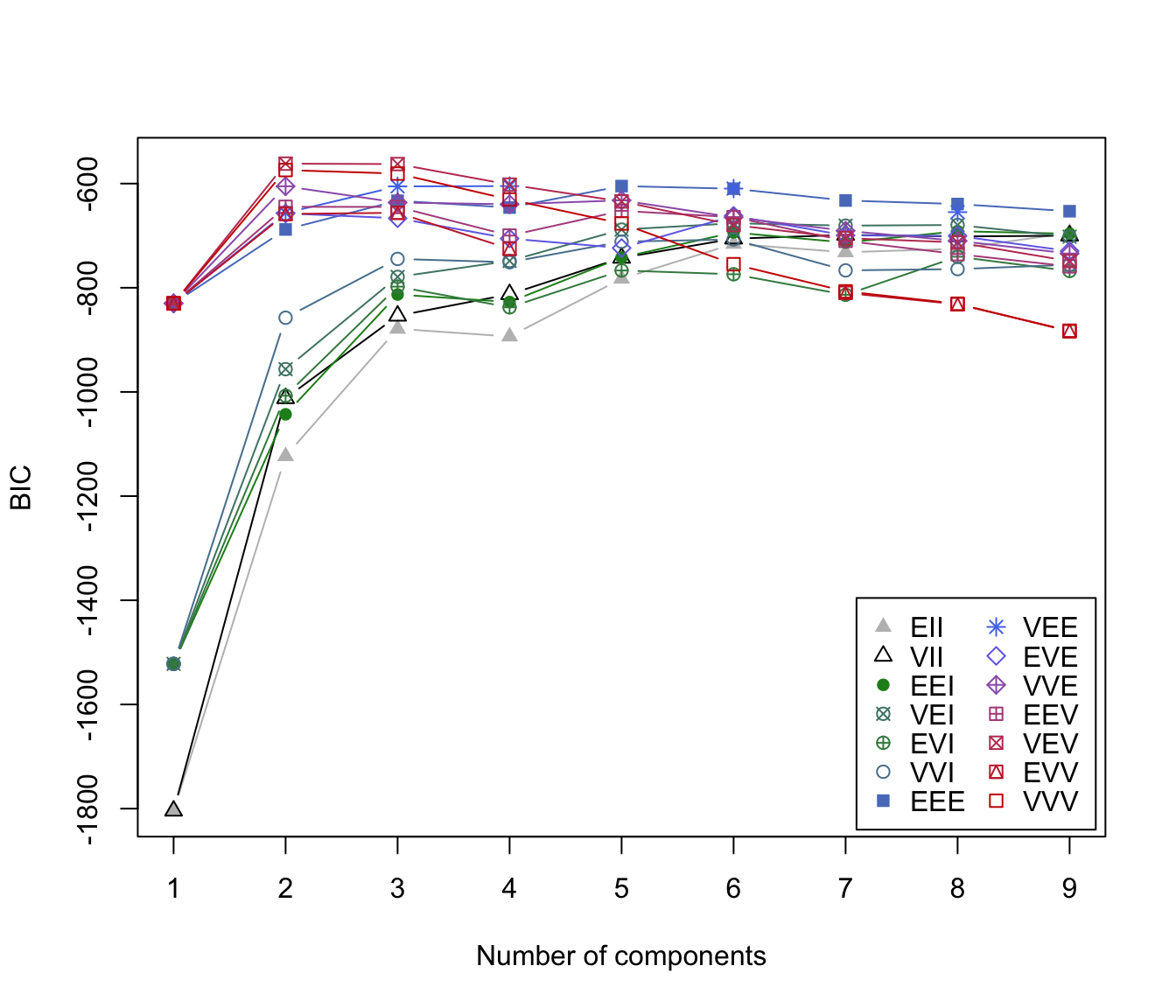Plots the BIC values returned by the mclustBIC function.

# S3 method for mclustBIC
plot(x, G = NULL, modelNames = NULL,
symbols = NULL, colors = NULL,
xlab = NULL, ylab = "BIC",
legendArgs = list(x = "bottomright", ncol = 2, cex = 1, inset = 0.01),
...)

Arguments

x

Output from mclustBIC.

G

One or more numbers of components corresponding to models fit in x. The default is to plot the BIC for all of the numbers of components fit.

modelNames

One or more model names corresponding to models fit in x. The default is to plot the BIC for all of the models fit.

symbols

Either an integer or character vector assigning a plotting symbol to each unique class in classification. Elements in colors correspond to classes in order of appearance in the sequence of observations (the order used by the function unique). The default is given by mclust.options("classPlotSymbols").

colors

Either an integer or character vector assigning a color to each unique class in classification. Elements in colors correspond to classes in order of appearance in the sequence of observations (the order used by the function unique). The default is given by mclust.options("classPlotColors").

xlab

Optional label for the horizontal axis of the BIC plot.

ylab

Label for the vertical axis of the BIC plot.

legendArgs

Arguments to pass to the legend function. Set to NULL for no legend.

...

Other graphics parameters.

Value

A plot of the BIC values.